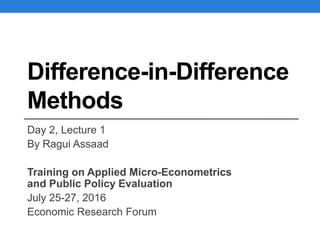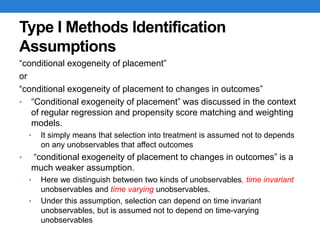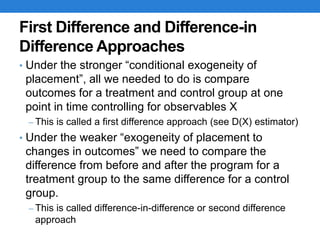Successfully reported this slideshow.

# Difference-in-Difference Methods×

# Difference-in-Difference Methods

Caroline Krafft- ST. Catherine University

ERF Training on Applied Micro-Econometrics and Public Policy Evaluation
Cairo, Egypt July 25-27, 2016

www.erf.org.eg

Caroline Krafft- ST. Catherine University

ERF Training on Applied Micro-Econometrics and Public Policy Evaluation
Cairo, Egypt July 25-27, 2016

www.erf.org.eg

### Difference-in-Difference Methods

1. 1. Difference-in-Difference Methods Day 2, Lecture 1 By Ragui Assaad Training on Applied Micro-Econometrics and Public Policy Evaluation July 25-27, 2016 Economic Research Forum
2. 2. Type I Methods Identification Assumptions “conditional exogeneity of placement” or “conditional exogeneity of placement to changes in outcomes” • “Conditional exogeneity of placement” was discussed in the context of regular regression and propensity score matching and weighting models. • It simply means that selection into treatment is assumed not to depends on any unobservables that affect outcomes • “conditional exogeneity of placement to changes in outcomes” is a much weaker assumption. • Here we distinguish between two kinds of unobservables, time invariant unobservables and time varying unobservables. • Under this assumption, selection can depend on time invariant unobservables, but is assumed not to depend on time-varying unobservables
3. 3. First Difference and Difference-in Difference Approaches • Under the stronger “conditional exogeneity of placement”, all we needed to do is compare outcomes for a treatment and control group at one point in time controlling for observables X – This is called a first difference approach (see D(X) estimator) • Under the weaker “exogeneity of placement to changes in outcomes” we need to compare the difference from before and after the program for a treatment group to the same difference for a control group. – This is called difference-in-difference or second difference approach
4. 4. The Identification Assumption Graphically
5. 5. The Difference-in-Difference Estimator DD(X ) = E(Y1 T -Y0 T | X ,T1 =1)- E(Y1 C -Y0 C | X,T1 = 0) If identifying assumption is met, then DD(X) = E(G1 | X ,T1 =1) = ATT(X ) If the counterfactual expectations are not expected to change over time, then the term on the right is zero E(Y1 C -Y0 C | X,T1 =1) = 0 Under such an assumption, the DiD estimator reduces to the Before and After (BA) estimator on the treated group, making measurement on a comparison group unnecessary BA(X ) = E(Y1 T -Y0 T | X,T1 =1) Such an assumption is unlikely to hold in practice.. Gives
6. 6. Data Structures for DiD Estimation When we have multiple observations on the same units, we effectively have panel data • Panel data can come in a number of different shapes • Let’s talk about shapes with a person-time period structure (say, before and after) • “Long” data: an individual shows up twice, once in time period 0 and once in time period 1. An observation is an individual in a time period • “Wide” data: an observation is a person, and there are different variables for each time period
7. 7. Notation for data shapes (in STATA) • i is a unique ‘logical observation’ (like a person, who may then be observed over time) • j is a ‘sub-observation’ (like a time period—people are observed in time periods) • x_ij are variables that can change over i and j (people and time), like employment status • Some variables are ‘time-varying,’ and can change over time (i.e. income or employment status) • Some variables are ‘time invariant’ and cannot change over time (i.e. sex) • Data entry errors or other issues may make time invariant variables time-varying in actual data 7
8. 8. Long Data: An Example • An observation is a person (id=i) in a year(=j) • A person has multiple observations (multiple years) • inc (income) is time varying (x_ij) • Sex is time invariant 8
9. 9. Wide data: an example • An observation is a person (id=i) • A person has only one observation • There is still only one variable for sex (time invariant) • Now there are three variables for income • One for each year • There is no more year variable—it is a suffix on income 9
10. 10. Reshaping Data from Long to Wide and vice versa • You can use the “reshape” command in STATA to reshape data from long to wide and vice versa. • However before you do that, you need to know what form your data is in. • The “duplicate report” command on the unique individual identifier can allow you to do that.
11. 11. Estimating the DD Estimator in Practice: The Regression Approach • Shape the data up as “long”, with each observation being an individual i time period t • Run a regression of the outcome (Yit) on the treatment status (Ti1), a time dummy (t) (t=0, before, t=1, after) (Ti0 = 0, by definition) Yit =a +bTi1 t +gTi1 +dt +eit Calculate the following expectations: E(Yi0 |Ti1 = 0) =a E(Yi1 |Ti1 = 0) =a +d E(Yi0 |Ti1 =1) =a +g Note: We can ignore the X’s since this regression does not et have covariates. I also dropped the T and C superscripts since we only observed treatment for treated observations and non-treatment for comparison observations E(Yi1 |Ti1 =1) =a +b +g +d
12. 12. SD1 = E(Yi1 |Ti1 =1)- E(Yi1 |Ti1 = 0) = (a +b +g +d)-(a +d) = b +g SD0 = E(Yi0 |Ti1 =1)- E(Yi0 |Ti1 = 0) = (a +g)-(a) =g Estimating the DD Estimator in Practice: The Regression Approach The Single Difference Estimator after Treatment is given by: The Single Difference Estimator before Treatment is given by: You can think of this estimator as measuring the effect of selection – the difference between treatment and comparisons observations before any treatment is applied.
13. 13. • The Difference-in-Difference Estimator or the Double Difference estimators is given by: Estimating the DD Estimator in Practice: The Regression Approach DD = SD1 -SD0 = b Thus, under the weaker Type I identification assumptions, the effect of the treatment on the treated (ATT) is given by the regression coefficient β, which is the coefficient of the interaction term between the treatment dummy and the time dummy. SD1 is an unbiased measured of ATT if and only if ϒ=0, which means there is no selection.
14. 14. Introducing Covariates • It is easy to introduce covariates in DiD estimation Yit =a +bTi1 t +gTi1 +dt + qj Xitj j å +eit All the expectations can now be written given X. ATT is still equal to β. To obtain heterogeneous effects, all you ned to do is interact β & ϒ with the Xs.
15. 15. An Example: Wahba and Assaad (2014). Flexible Labor Regulations and Informality in Egypt • Studies the effects of the 2003/04 labor law on informality of employment in Egypt • Research Design • Two observation periods • 1998-2002 pre-intervention P=0 (P=post) • 2004-2008 post-intervention P=1 • Two groups of private non-agricultural wage workers who would be differentially affected are observed over a 5-yer period, either pre- or post-intervention • Workers initially non-contracted working for formal/semiformal enterprises (F) (T=1) • Workers initially non-contracted working in informal enterprises (I) (T=0) • Contention is that second group of workers unlikely to be affected by new labor law
16. 16. Wahba and Assaad (2014) • Estimation Equation Where: Cit is a dummy indicating the contract status of the worker Tit is a dummy indicating belonging to F (versus i) Pit is a dummy indicating post Xit is a vector of observables including individual characteristics, such as gender, education and region, GDP growth rates, annual unemployment rates, a time trend variable capturing the time since the job started We posit that δ captures he effect of the law. Cit =a +bTit +g Pit +dTit Pit +jXit +eit
17. 17. Difference-in-Difference Estimates of the Determinants of Acquiring a Job Contract α α+β β α+ϒ α+β+ϒ+δ β+δ δ
18. 18. Falsification Tests • The identifying assumption for DiD means that the trend in the outcome variable between treated and control observations (conditional on observables) should not be different absent treatment. • To check on this, one can check whether the trend is the same in the pre-treatment period. • To do this, you will need two points in time prior to treatment begins. • The same regression is carried out for that pre-treatment period. The coefficient β needs to be statistically insignificant in this case to pass the falsification test.
19. 19. Cross-Sectional Difference-in- Difference • DiD estimators do not have to involve measurements across time. • Can be done across groups of individuals, with one group not expected to be affected by treatment. • E.g. • Target and non-target villages for micro-credit programs • Participating and non-participating households • Non-targeted villages are not expected to be affected, so difference between participating and non-participating HH in such villages could be a measure of selection into participation • DiD: difference between participants and non-participants in targeted villages, minus the same difference in non-targeted villages
20. 20. Difference-in-Difference and PSM • One can use DiD in the context of PSM as well • Matching is still done along pre-treatment characteristics ATT =(1/NT ) [(Y1 j T -Y0 j t ) j=1 NT å - wij iÎC å (Y1ij C -Y0ij C )] Using “wide” data, calculate Y1j - Y0j at the individual level and then carry out PSM on that outcome variable
21. 21. Wahba and Assaad (2014) PSM Robustness check: • Treatment: (as before) is belonging to a formal firm or informal firm at the beginning of the perio T=1 in formal firm T=0 in informal firm • Outcome: whether individual with no contract in 2002-2004 moved to a contract job in 2004-2008. Y=1 obtained contract Y=0 did not obtain contract • Falsification test • Do it again for period 1996-98 to 1998-2002
22. 22. Wahba and Assaad (2014) Coefficient (DiD) Bootstrapped Std Err Post Policy (2004-2008) 0.0330** 0.0174 Pre Policy (1998-2002) 0.0043 0.0145 Covariates used in matching: Gender, Age, Education, Region of Residence Conclusion: • The proportion who moved from no contract to a contracts in Formal Firms exceeds that of in Informal Firms by 3.3 percentage points • No such difference can be seen in the pre-policy period